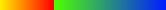# Custom palette color

Hi!

I’d like to make a custom color palette for a TH2D, so that the color suddenly changes above a spefic value.

I think it is easier for me to explain what I need by using a picture:

(this picture comes from: https://root.cern.ch/rainbow-color-map)

In that picture the color changes abruptly at z = 0.

I need something similar at a different value ( z = 1.5 ).

This is what I can do by now:

``````TH2D * c () {
gStyle->SetOptStat(0);
Double_t x, y;

Int_t NCont = 800;
Double_t R[] = {0.00, 0.00, 1.00};
Double_t G[] = {1.00, 0.00, 0.00};
Double_t B[] = {0.00, 1.00, 0.00};
Double_t s[] = {0.00, 0.25, 1.00};
TColor::CreateGradientColorTable(3, s, R, G, B, NCont);
gStyle->SetNumberContours(NCont);

TH2D * c = new TH2D("h", "test", 50, 0, 1, 50, 0, 1);
for (Int_t i = 0; i < 50000; i++) {
x = gRandom->Gaus(0.5, 0.2);
y = gRandom->Gaus(0.5, 0.2);
c->Fill(x, y);
}

c->Draw("colz");
return c;
}
``````

``````{
TH2F *h1 = new TH2F("h1","h1",100,-4,4,100,-4,4);
h1->SetStats(0);

Double_t a,b;
for (Int_t i=0;i<50000;i++) {
gRandom->Rannor(a,b);
h1->Fill(a-1.5,b-1.5,0.08);
}

Double_t max       = h1->GetMaximum();
Double_t min       = h1->GetMinimum();
Double_t val_white = 0.5;
Double_t per_white = (val_white-min)/(max-min);

const Int_t Number = 3;
Double_t Red[Number]   = { 0., 1., 1.};
Double_t Green[Number] = { 0., 1., 0.};
Double_t Blue[Number]  = { 1., 1., 0.};
Double_t Stops[Number] = { 0., per_white, 1. };

Int_t nb= 256;
h1->SetContour(nb);

h1->Draw("colz");
}
``````

Uhm… No, it’s not what I need.

There’s still same smooth color change there, from blu to white and then from white to red.

I need a discontinuity, like here, from red to green:``````{
TH2F *h1 = new TH2F("h1","h1",100,-4,4,100,-4,4);
h1->SetStats(0);

Double_t a,b;
for (Int_t i=0;i<50000;i++) {
gRandom->Rannor(a,b);
h1->Fill(a-1.5,b-1.5,0.08);
}

Double_t max     = h1->GetMaximum();
Double_t min     = h1->GetMinimum();
Double_t val_cut = 0.5;
Double_t per_cut = (val_cut-min)/(max-min);

const Int_t Number = 4;
Double_t Red[Number]   = { 0., 1., 0., 1.};
Double_t Green[Number] = { 0., 0., 1., 0.};
Double_t Blue[Number]  = { 1., 1., 0., 0.};
Double_t Stops[Number] = { 0., per_cut, per_cut+0.01, 1. };

Int_t nb= 256;
h1->SetContour(nb);

h1->Draw("colz");
}
``````

This looks like cheating, but ok.Thank you!

Why cheating ? … we simply make the gradient change very quickly.
Make epsilon = 0.0001 … it will look better.

Well…But it’s ok anyway. I had in mind a similar solution but I thought there had to be a direct/polish way to get what I needed.It works, and it works quite fine, so I’m ok with that. It’s just that I thought there was a specific method for that.

It’s quite direct seems to me.

This topic was automatically closed 14 days after the last reply. New replies are no longer allowed.